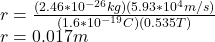## A singly charged positive ion has a mass of 2.46 ✕ 10−26 kg. After being accelerated through a potential difference of 270 V, the ion enters

Question

A singly charged positive ion has a mass of 2.46 ✕ 10−26 kg. After being accelerated through a potential difference of 270 V, the ion enters a magnetic field of 0.535 T, in a direction perpendicular to the field. Calculate the radius of the path of the ion in the field.

in progress 0
2 months 2021-09-01T07:30:48+00:00 1 Answers 4 views 0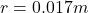Explanation:

Given data

Mass m=2.46×10⁻²⁶kg

Voltage V=270 V

Magnetic field B=0.5353 T

To find

Solution

By using conservation of energy to find speed of charge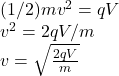Substitute the given values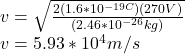So the radius can be find as: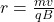Substitute the values